# A Primer on Latin Squares With Some Research Objectives

Deviating from my usual content, in this post I’m going to provide some necessary knowledge for working with a combinatorial object known as a Latin square. These objects were the centerpiece of my (IMO poorly written) undergraduate thesis and are one focal point of research that I continue to this day, development of this research can be seen on its GitHub page. This post can be considered a primer for understanding other posts pertaining to the research I’m doing with these objects which is largely computational and, at this time, includes developing more efficient algorithms and computing paradigms for generating Latin squares and determining if groups of Latin squares have a specific property known as (mutual) orthogonality (a glimpse into that can be seen in a past blog post of mine, although this code has changed since then). Information about Latin squares, Latin square structures, and Latin square properties pertinent to the development of these algorithms and my ongoing research are provided below.

## Latin Squares

A Latin square of order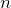is a square array that containsdifferent elements all occurringtimes but with none occurring more than once in the same row or column. That’s a little wordy, it’s much easier to see an example of a Latin square. Below is a Latin square of order 4.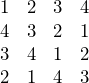As seen here, the symbols 1, 2, 3, and 4 each occur 4 times but not more than once in any row or column. One good “real-world” example of a Latin square is a completed Sudoku puzzle which is an order 9 Latin square. The only difference is that the rule of the 3 by 3 subsquares in a Sudoku puzzle that also must contain the numbers 1 thru 9 is not applicable in the Latin square.

One of the primary uses of Latin squares outside of games is in statistics and the design of experiments via Latin square design. Other applications include error-correcting codes, the construction of Cayley tables in Algebra, and other mathematical games and puzzles. More information on these applications can be found on the Latin square Wikipedia page.

### Normalized Latin Squares

Below are two more examples of Latin squares, the first of order 3 and the second of order 5. Notice that, unlike the square above, the first row and column of the squares are in ascending numerical order.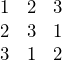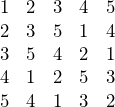Latin squares taking this form are called normalized Latin squares. More formally, a normalized Latin square is a Latin square in which the first row and column are given by 1, 2, …, n. Any Latin square can be normalized by applying a few operations, namely row and column permutations. For example, the order 4 square given above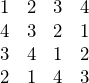can be normalized by simply swapping the second and fourth rows. Doing so produces the normalized square below.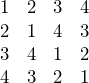Typically more row and column permutations are necessary to normalize a Latin square but it is easily done nonetheless.

### “Reduced” Latin Squares

Although this terminology seems to be non-standard, I was taught that a reduced Latin square is one in which the first row is given by 1, 2, …, n. That is, a reduced Latin square is almost a normalized Latin square but the first column need not be in ascending numerical order. The first order 4 square presented in this post is a reduced Latin square.On the Latin square Wikipedia page, and most other sources, reduced is treated as synonymous with normalized so that is probably more conventional. I can’t find a better name for these squares, I’m not sure if one even exists, but this particular structure is very important in determining Latin square orthogonality since, rather than checking every square of order n for orthogonality, only the reduced squares of order n need to be checked.

### Isotopy Classes

Two Latin squares belong to the same isotopy class if one square can be obtained from the other by permuting the rows, columns, and symbols of the other square. More simply, given the three operations of swapping rows, swapping columns, and swapping symbols (e.g. all 3’s become 1’s and all 1’s become 3’s) if, when applying these three operations, we end up with a new Latin square than the newly created Latin square is in the same isotopy class as the first. Two Latin squares are said to be isotopic or to have isotopy class equivalence if they belong to the same isotopy class.

There are a set of squares for each order known as isotopy class representatives. Recursively permuting the rows, columns, and symbols of an isotopy class representative produces all of the squares in that isotopy class. That is, the rows, columns, and symbols of the isotopy class representative are permuted to create a set of new squares. The rows, columns, and symbols of these new squares are then also permuted to create yet more new squares. This is done until no new squares are generated. Permuting the rows, columns, and symbols of all isotopy class representatives in this way produces all of the Latin squares of a particular order.

For order 5 there are two isotopy classes. The isotopy class representatives for these two classes are given below.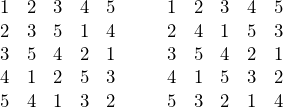By way of example, we can generate a new square by first permuting (swapping) the rows, we’ll do rows one and two of the leftmost isotopy class representative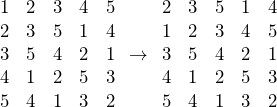This operation in and of itself creates a new Latin square. However, we can continue to swap rows, columns, and symbols to create even more squares. Taking the newly generated square above and swapping columns 3 and 4 creates another new square.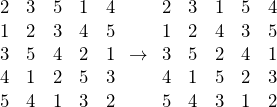To show an example of symbol permutation the original isotopy class representative will have the symbol “3” swapped with the symbol “1”. That is, this operation entails switching all 3’s in the square to 1’s and vice versa. Again, this produces a new, distinct Latin square.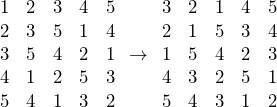Isotopy classes are important because they allow us to generate all Latin squares of a particular order given just a few squares of that order, i.e. one from each isotopy class. For example, there are 22 isotopy classes of order 6. Thus, given just 22 Latin squares of order 6, the rows, columns, and symbols can be permuted to generate the remaining 812,851,178 squares (there are 812,851,200 total squares of order 6).

### (Mutually) Orthogonal Latin Squares

Latin square orthogonality was the focal point of my undergraduate thesis. Two distinct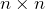Latin squares,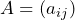and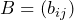, are said to be orthogonal if the ordered pairs (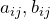) are all distinct, here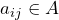and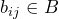are the elements of the Latin squares at row i and column j. A set of Latin squares each orthogonal to one another is called mutually orthogonal, and the squares are mutually orthogonal Latin squares (MOLS). This property, like many of the others, is most easily understood via example. Below is a set of two order 4 Latin squares which are orthogonal.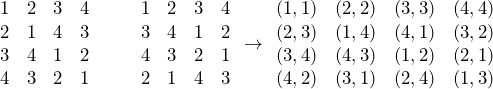The square of pairs is constructed by taking the element at position (i,j) in the two Latin squares and creating the pair () in the rightmost square. It can easily be verified that none of the pairs in the resulting square are repeated, therefore these two Latin squares are orthogonal. Contrarily,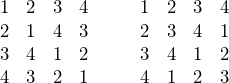are not orthogonal because after constructing the square containing the pairs of entries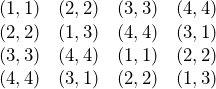it can be seen that the pairs (1,1), (2,2), (3,3), (4,4), (1,3), and (3,1) are all repeated. The three squares below are mutually orthogonal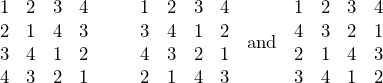Verification of the orthogonality of these squares is omitted due to the length of this post.

Orthogonal Latin squares are used in a variety of applications. For example, they are used for the design of experiments, in error-correcting codes, and in determining the existence of another combinatorial object known as a finite projective plane. The last application was devised by R. C. Bose in the following theorem:

Theorem: A finite projective plane of order n exists if and only if there exists a complete set of MOLS, i.e. a set of MOLS containing (n-1) Latin squares of order n.

## Current Research

My undergraduate research consisted of studying Latin squares. Having never taken a combinatorics course, the “research” began as a self-study of these objects. Eventually, I was tasked with determining the orthogonality of Latin squares of orders 2-6. Interestingly, while looking at the isotopy classes that the orthogonal Latin squares “came from” it was determined that all of the orthogonal squares were also isotopic. Furthermore, the isotopy class representative of this isotopy class happened to be symmetric. Exploring this further it was shown to be true for order 5, trivially true for order 4 (all isotopy class representatives are symmetric), and vacuously true for order 6 (there are no orthogonal squares of order 6). Initially, the continuation of my research consisted of determining if this conjecture was true for higher orders, i.e. are orthogonal Latin squares isotopic to a symmetric Latin square. To continue to study this for higher-order Latin squares, efficient algorithms to handle large numbers of Latin squares needed to be created.

Currently, the most memory-efficient way to generate Latin squares is to generate one isotopy class at a time. This way, after no new squares are generated for an isotopy class, the structures storing the Latin squares, and any metadata for their generation, can be cleared and the memory can be freed. However, I’m currently working on a GPGPU algorithm that generates the squares en masse and disregards the isotopy classes. Doing it this way uses a good chunk of memory which my desktop can’t handle (32 GB of RAM). The problem arises from the need to keep a list of all of the distinct previously generated squares. This is done to determine if any newly generated squares have already been created, the squares are discarded if so. More information about the algorithms used to generate Latin squares can be found in my undergraduate thesis.

To consider the memory implications assume we’re generating Latin squares of order 6, therefore we need to store 36 values ranging from 0 to 5. This can be done optimally with 3 bits (in fact, 3 bits would get us all the way through order 8). So, to store a single Latin square of order 6, 108 bits will be used. In practice, doing bitwise operations to store each digit in 3 bits of, say, a 64-bit integer is tedious so realistically small data types, like short in C++, are used to store the squares’ values. Therefore, each square realistically requires at least 576 = 36×16 bits for storage. However, there are only approx. 812 million Latin squares of order 6. A 32-bit integer can easily represent all 812 million values. Thus, if a mapping could be found that takes the Latin square of order n to a distinct real value the algorithm would use less than one-third of the optimal memory and approx. 6% of the memory of a realistic implementation for order 6. This efficiency would increase further for higher-order Latin squares. Finding a mapping,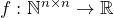, is, currently, one of my primary research objects. Note that, the mapping need not preserve which square is represented by which real value; we only care whether or not a square was generated not what the square actually was.

Along the way, I’ve also built an interest in another open problem with orthogonal Latin squares: is there a set of 3 mutually orthogonal Latin squares of order 10? It has been shown that there are multiple couples of Latin squares of order 10 and that there isn’t a family, i.e. a set of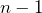(9 in this case) mutually orthogonal Latin squares, but the question as to the largest set of mutually orthogonal Latin squares of order 10 remains unsolved. Good results from my other activities in dealing with Latin squares could certainly help to answer this question.